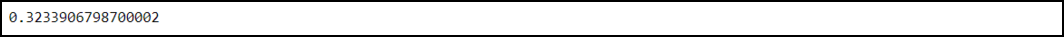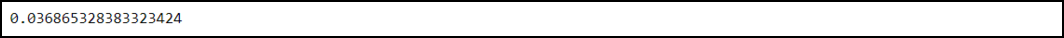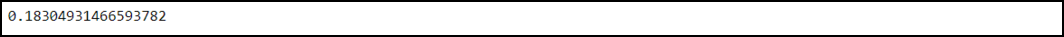GFG App
Open AppBrowser
Continue

# How to Find a P-Value from a t-Score in Python?

T-score: T-score is defined as the count of standard deviations from the mean data of a t-distribution. In simple words, it is defined as the ratio of the difference between the two-data groups and the difference between within the data groups. T-score is a statistical term and it is mainly used for the following things:

• Determine the upper and lower bounds of a confidence interval (For the approximately normally distributed data).
• Determine the p-value of the t-test and regression tests.

P-value: It defines the probability of the result taking place from the sample space by chance. P-value varies from 0 to 100%. Note that a lower p-value is considered good as it implies that a result didn’t take place by chance.

## Finding a p-value:

Syntax to install scipy library in python:

`pip3 install scipy`

Scipy is a python library used for scientific computation. It provides us scipy.stats.t.sf() function to compute the p-value.

scipy.stats.t.sf() function:

Syntax:

scipy.stats.t.sf(abs(t_score), df=degree_of_freedom

Parameters:

• t_score: It signifies the t-score
• degree_of_freedom: It signifies the degrees of freedom

The p-value is often linked with the t-score. We will now discuss how to calculate the p-value linked with the t-score for the left-tailed, right-tailed, and two-tailed tests.

## P-value in a left-tailed test:

In this program, the t score is -0.47, and the degree of freedom is equal to 12.

Example:

## Python3

 `# Python program to find the p-value  ` `# in a left-tailed test ` ` `  `# Importing the library ` `import` `scipy.stats ` ` `  `# Determine the p-value ` `scipy.stats.t.sf(``abs``(``-``.``47``), df``=``12``) `

Output:P-value in a left-tailed test

Hence, the p-value comes out to be equal to 0.32. If we use a significance level of α = 0.05, we will fail to reject the null hypothesis of our hypothesis test because here the p-value is greater than 0.05.

## P-value in the right-tailed test:

In this program, the t score is 1.87, and the degree of freedom is equal to 24.

Example:

## Python3

 `# Importing scipy library ` `import` `scipy.stats ` ` `  `# Determining the p-value ` `scipy.stats.t.sf(``abs``(``1.87``), df``=``24``) `

Output:P-value in the right-tailed test

Hence, the p-value comes out to be equal to 0.036. If we use a significance level of α = 0.05, we will have to reject the null hypothesis of our hypothesis test because here the p-value is less than 0.05.

## P-value in the two-tailed test:

In this program, the t score is 1.36, and the degree of freedom is equal to 33. Note that to find a two-tailed test p-value we simply multiply the p-value of the one-tailed p-value by two.

Example:

## Python3

 `import` `scipy.stats ` ` `  `# find p-value for two-tailed test ` `scipy.stats.t.sf(``abs``(``1.36``), df``=``33``)``*``2`

Output:P-value in the two-tailed test

Hence, the p-value comes out to be equal to 0.183. If we use a significance level of α = 0.05, we will fail to reject the null hypothesis of our hypothesis test because here the p-value is greater than 0.05.

My Personal Notes arrow_drop_up